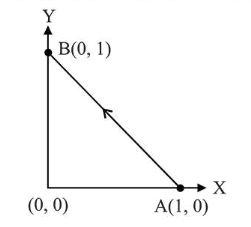# Solve this`
Question:

Consider a force $\overrightarrow{\mathrm{F}}=-x \hat{i}+y \hat{j}$. The work done by this force in moving a particle from point $A(1,0)$ to $B(0,1)$ along the line segment is: (all quantities are in SI units)1. 2

2. $\frac{1}{2}$

3. 1

4. $\frac{3}{2}$

Correct Option: , 3

Solution:

(3) Work done, $W=\int \vec{F} \cdot \overrightarrow{d s}$